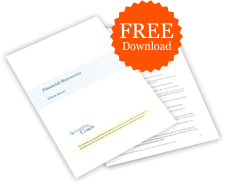# If cash and a note are exchanged for a plant asset, is the amount of the note used in the depreciation calculation?

A plant asset's cost is depreciated, unless the asset is land.

Cost is defined as the cash or cash equivalent amount at the time of the transaction. This means that the asset's cost is the cash amount plus the note's present value at time that the asset is purchased.

To illustrate this, let's assume that equipment is purchased by giving \$50,000 of cash plus a promissory note of \$100,000. If the note has an interest rate that is a fair rate considering the market rates and the riskiness of the party signing the note, then the present value of the note is \$100,000. The equipment will then be recorded at its cost of \$150,000. This cost of \$150,000 will be depreciated over the equipment's useful life.

If the note specifies zero interest, then the present value of the note is less than \$100,000. Let's assume that the note's present value is computed to be \$90,000. This means that the asset's cost will be \$140,000—the cash of \$50,000 plus the note's \$90,000 of present value. Assuming no salvage value, the total depreciation expense over the life of the equipment will equal \$140,000. The \$10,000 difference will be reported as interest expense over the life of the note.### Free Financial Statements Cheat Sheet

546,292
Subscribers
You are already subscribed. This offer is not available to existing subscribers.
Error: You have unsubscribed from this list.